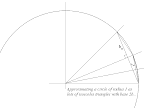## August 15, 2008

### Calculating Pi to 8 decimal places using Pythagoras's Theorem

"BORINGNESS WARNING [The following entry is of no interest to anyone who "can't do maths"]

I'm pretty bored. It's been raining on and off, but mainly on, for the last week in Shimla. There's nothing to do here when it rains except stay in the hotel and read. Other than computer manuals, I've been reading A Passage to India and also The Joy of Numbers, which I found in the second hand bookshop. The maths book is by someone called Shankuntala Devi who was a celebrity mathematician in India during the 80s. According to the book, Archimedes declared Pi to have a value between 3 1/7 and 3 10/7.
Monumentally bored of exam revision, I decided to see if I could calculate Pi myself. Approximating a circle as being composed of 'n' isosceles triangles, I found that:
pi=n*sin(180/n)
which is very accurate for a large values of n. It occurred to me that if Archimedes tried to derive pi on his own, he probably didn't know how to calculate 'sin', so I tried to come up with an expression for 'sin'. I could remember seeing an iterative equation for 'sin' at university, but I couldn't remember what it was and after several hours gave up trying to work it out. I went to sleep that night feeling a little disappointed that I couldn't do ancient mathematics without sinning.
This morning I had another go at it, and decided to forget about 'sin' and use only Pythagoras's. Theorem. After about eight pages of scribbling I solved it:
pi=n*bn
where bn is 0.5 * base of an isoceles triangle formed when approximating a circle of radius 1 as n triangles, and
bn=0.5*sqrt[2-(2*sqrt[1-bn-12]]
Using n=4 as a starting point (a square bounded by a circle) and b=sqrt (from simple geometry) I managed to get pi=3.14 after 4 iterations:
`1st iteration - n=4, b=sqrt2, pi=2.822nd iteration - n=8, b=0.38, pi=3.063rd iteration - n=16, b=0.2, pi=3.124th iteration - n=32, b=0.1, pi=3.14`Enlarge diagram (in new window)

So, a circle modelled as 32 isoceles triangles gives pi=3.14. This requires quite a lot of paper to work out all the intermediate calulations (especially if you have to calculate all the square roots by hand - I used Windows calculator instead).
I used a spreadsheet to continue the calculations. This gives, for a circle consisting of 32768 triangles, pi= 3.14159265 which is accurate to 8 decimal places.
According to The Joy of Numbers, Ludolph van Ceulen, a german presumably, calculated pi to 35 decimal places in the 16th century without a spreadsheet. He presumably used a more elegant mathematical method, but it would still have taken a ridiculous degree of patience to do accurately. Perhaps he was in Shimla at the time.